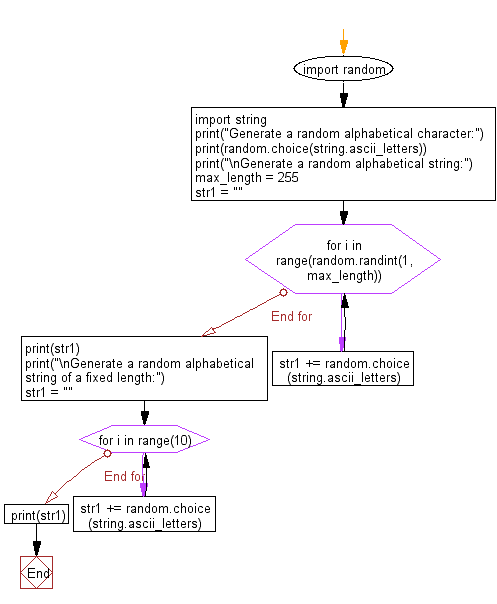﻿ Python: Generate a random alphabetical character, string and alphabetical string of a fixed length - w3resource# Python: Generate a random alphabetical character, string and alphabetical string of a fixed length

## Python module: Exercise-3 with Solution

Write a Python program to generate a random alphabetical character, alphabetical string and alphabetical string of a fixed length.

Use random.choice

Sample Solution:

Python Code:

``````import random
import string
print("Generate a random alphabetical character:")
print(random.choice(string.ascii_letters))
print("\nGenerate a random alphabetical string:")
max_length = 255
str1 = ""
for i in range(random.randint(1, max_length)):
str1 += random.choice(string.ascii_letters)
print(str1)
print("\nGenerate a random alphabetical string of a fixed length:")
str1 = ""
for i in range(10):
str1 += random.choice(string.ascii_letters)
print(str1)
```
```

Sample Output:

```Generate a random alphabetical character:
e

Generate a random alphabetical string:
wxEGKdCBCkJZrFscEDXhxAovbTkPzlfCxRQCMbuvquFXyFHivyEeqNGzeWxlKZiFzVIuyKLEtPJHbvqQTpIJoMTPFUbrQjGEfXRTlQNKviduRaNDdtEbExXhdbLKiIprgdYTivZDFjk

Generate a random alphabetical string of a fixed length:
ijdvKiSWwO
```

Flowchart:## Visualize Python code execution:

The following tool visualize what the computer is doing step-by-step as it executes the said program:

Python Code Editor:

Have another way to solve this solution? Contribute your code (and comments) through Disqus.

What is the difficulty level of this exercise?

Test your Python skills with w3resource's quiz

﻿

## Python: Tips of the Day

For-else construct useful when searched for something and find it:

```# For example assume that I need to search through a list and process each item until a flag item is found and
# then stop processing. If the flag item is missing then an exception needs to be raised.

for i in mylist:
if i == theflag:
break
process(i)
else:
raise ValueError("List argument missing terminal flag.")
```